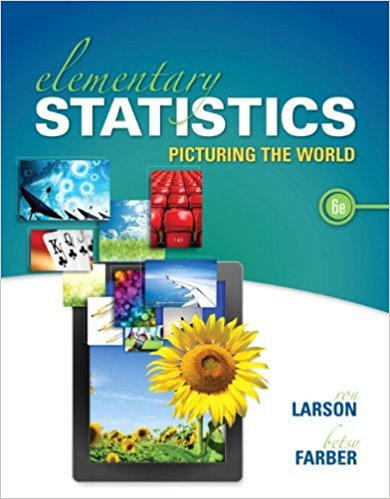×
Get Full Access to Elementary Statistics: Picturing The World - 6 Edition - Chapter 4.3 - Problem 22e
Get Full Access to Elementary Statistics: Picturing The World - 6 Edition - Chapter 4.3 - Problem 22e

×

# Using a Distribution to Find Probabilities In | Ch 4.3 - 22EISBN: 9780321911216 66

## Solution for problem 22E Chapter 4.3

Elementary Statistics: Picturing the World | 6th Edition

• Textbook Solutions
• 2901 Step-by-step solutions solved by professors and subject experts
• Get 24/7 help from StudySoup virtual teaching assistantsElementary Statistics: Picturing the World | 6th Edition

4 5 1 369 Reviews
16
2
Problem 22E

Problem 22E

Using a Distribution to Find Probabilities In Exercise, find the indicated probabilities using the geometric distribution, the Poisson distribution, or the binomial distribution. Then determine whether the events are unusual. If convenient, use a table or technology to find the probabilities.

Breaking Up Twenty-nine percent of Americans ages 16 to 21 years old say that they would break up with their boyfriend or girlfriend for \$10,000. You randomly select seven 16- to 21-year-olds. Find the probability that the number of 16- to 21-year-olds who say that they would break up with their boyfriend or girlfriend for \$10,000 is (a) exactly two, (b) more than three, and (c) between one and four, inclusive.

Step-by-Step Solution:

Step 1 of 1

(a)

We are asked to find the indicated probabilities using the geometric distribution, the Poisson distribution, or the binomial distribution.

Then determine whether the events are unusual.

If convenient, use a table or technology to find the probabilities.

We have given 29% of Americans ages 16 to 21 years old say that they would break up with their boyfriend or girlfriend for \$10,000.

You randomly select seven 16 to 21 years old.

We are asked to find the probabilities that the number of 16 to 21 years old who say that they would break up with their boyfriend or girlfriend for \$10,000.

1. Exactly two,
2. More than three,
3. Between one and four, inclusive.

The probability distribution would be the binomial distribution since the experiment has a fixed number n of independent trials.

A binomial distribution represented by the following formula,WhereWe need to find the exactly twoWe need to find more than threeWe need to find between one and four, inclusiveSince the value of probabilities are not less than 0.05, hence there are no events which are unusual.

Using Minitab, we can find the probabilities.

We need to find the exactly twoSteps to find out the pdf of the Binomial distribution.

1. Go to menu Calc under that select sub menu probability distributions.
2. Under probability distributions select a Binomial.
3. Click on radio button probability and enter the relevant data.We need to find the exactly twoWe need to find between one and four, inclusive(x) P(X=x) 1 0.260044 2 0.318645 3 0.216918 4 0.0886Step 2 of 1

##### ISBN: 9780321911216

Unlock Textbook Solution